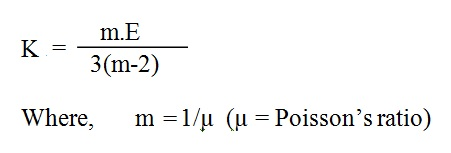# Mechanical Engineering

Basics of Mechanical Engineering

## LATEST

➦ The relation between Bulk Modulus (K) and Young's Modulus (E) is given by,➦ The relation between Modulus of Elasticity (E) and Modulus of Rigidity (C) is given by,➦ The relation between Modulus of Elasticity (E), Modulus of Rigidity (C) and Bulk Modulus (K) is given by,